# Regular Expressions and Packages in Python for Data Science

## Regular Expressions and Packages in Python for Data Science

In this article, I am going to discuss Regular Expressions and Packages in Python for Data Science with Examples. Please read our previous article where we discussed Numpy and Pandas with Matplotlib and Seaborn with Examples. At the end of this article, you will understand the following pointers.

1. The Sys Module
2. Interpreter Information
3. Launching External Programs
4. path directories and Filenames
5. Walking Directory Trees
6. Math Function
7. Random Numbers
8. Dates and Times
##### The Sys Module in Python

The sys module in Python affords diverse features and variables that can control one-of-a-kind elements of the Python runtime environment. Moreover, it permits working at the interpreter because it allows entry to the variables and features that interact strongly with the interpreter.

##### sys.version –

You can use the sys.version to fetch the model/version of Python Interpreter with some additional piece of information. This suggests how the sys module interacts with the interpreter.

###### Example –
```import sys

print(sys.version)
```
###### Output: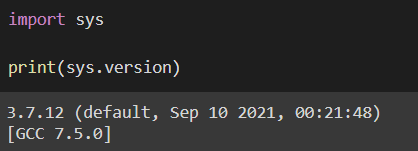##### sys.exit([arg]) –

It may be used to go out of the program. The non-obligatory argument arg may be an integer giving the go out or some other sort of object. If it’s far an integer, 0 is considered as empty memory.

###### Example –
```import sys

number = int(input("enter a number : "))

if number%2!=0:
# exits the program
sys.exit("Not an even number")
else:
print("This is an even number")
```
###### Output: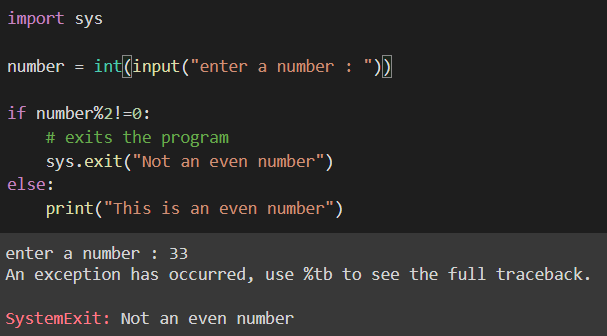##### sys.path –

The sys module’s built-in variable sys.path delivers a list of directories where the interpreter will look for the required module. When a module is imported into a Python file, the interpreter looks for it among the interpreter’s built-in modules first. Then, it searches the list of directories defined by sys.path if none are found.

###### Example –
```import sys

print(sys.path)
```
###### Output: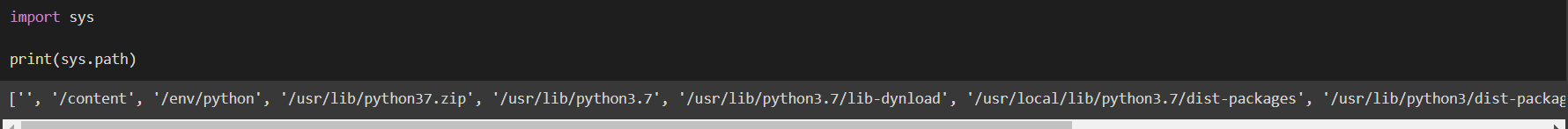##### Interpreter Information in Python

A program that runs other programs is known as an interpreter. When you build Python applications, the language turns the developer’s source code into an intermediate language, which is then translated into the native language/machine language that is executed.

Python code is compiled into byte code, which results in a file with the extension .pyc. Internal byte code compilation took place, virtually fully hidden from the developer. The compilation is merely a translation process, and byte code is a platform-independent, lower-level representation of your source code. Each of your source statements is roughly converted to a set of byte code instructions. This byte code translation is done to speed up the execution of the code. Byte code can be executed much faster than the original source code statements.

The .pyc file, which was created during the compilation process, is then executed by virtual machines. The Virtual Machine is nothing more than a huge loop that iterates over your byte code instructions one by one, performing their operations. The Virtual Machine is Python’s runtime engine, and it is the component that actually runs Python scripts. It is always present as part of the Python system. In technical terms, it’s just the final stage of the Python interpreter.

You can use the sys.version to fetch the model/version of Python Interpreter –

```import sys

print(sys.version)
```
###### Output:##### Launching External Programs in Python

External programs are very important in most programming languages, especially scripting languages like BASH and Python. External program execution can be divided into two categories: synchronous and asynchronous. The synchronous mode sends out external directives and then waits for a response. Asynchronous mode, on the other hand, immediately returns to the main thread.

There are numerous ways to run external programs in Python. Importing the os package is the simplest option. It includes the popen() and system() functions.

The output (stdout, stderr) will be treated as a file object by os.popen(), allowing you to capture the output of external programs. It’s an example of a synchronous method.

```import os
```
###### Output: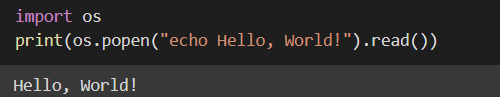The exit status is returned by the os.system(), which is also synchronous and pretty simple to use.

```import os
```
##### Path directories and Filenames in Python

Folders are what make up directories. You can find these directories within a root folder, for example, C:\ or D:\, and each one can contain files or subdirectories.

In order to get a file in Python, you must know its exact path. In Windows, you may inspect a file’s path by right-clicking it and selecting File-> Properties-> General-> Location.

Similarly, in order to start a script, the working directory must be set to the script’s location. However, the Current Working Directory (CWD) is critical when you run numerous scripts.

If the files aren’t in the current working directory, it won’t be possible for Python to access them. The Python ‘get current directory’ tool can help you to determine the directory you are currently working on.

##### Get the current directory in Python:

We utilize the OS module to interface with the operating system to retrieve the directory you are presently in. The os.getcwd() method in the OS module is used to return the current directory’s path.

```#importing the os module
import os

#to get the current working directory
directory = os.getcwd()

print(directory)
```
###### Output: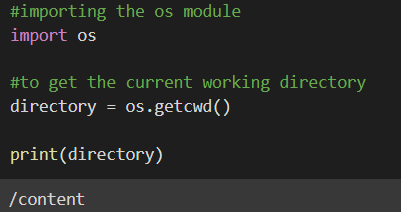##### Change directory in Python

We use the chdir() methods in the os module to change the current directory, similar to how we used the os.getcwd method in Python to get the current directory. To get files or run scripts from other folders, the current directory is altered.

```import os

os.chrdir("C:/Myfolder/sample")
```

Using Python, you can extract the file name directory from the file path by using multiple ways. You can use either of these –

##### Using os.path.basename()

You may alternatively get the filename from the path using a method supplied by the os.path library. The basename function is used to retrieve the file’s name. The filename is returned by the basename function, which takes a path as an input.

###### Example –
```import os

print(os.path.basename("C:/Myfolder/sample"))
```
###### Output: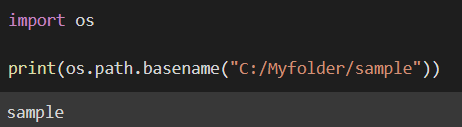##### Using os.path.split()

The os.path.split() method can be used if the head and tail of a path are required separately. This method accepts a path as an argument and returns the path’s head and tail.

###### Example –
```import os

print(tail)
```
###### Output: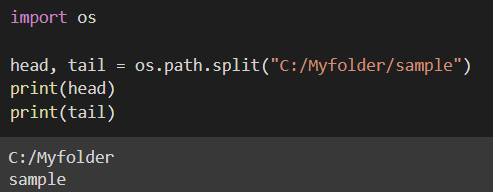##### Walking Directory Trees in Python

In Python, how do you explore a file system? Let’s say we have the following file structure in our system and we want to go through all of its branches from top to bottom.

os.walk() generates file names in a directory tree by walking top-down or bottom-up through it. It returns a 3-tuple for each directory in the tree rooted at the directory top (including top itself) (dirpath, dirnames, filenames).

1. root: Prints only the directories that you specify.
2. dirs: Prints subdirectories from the root directory.
3. files: Prints all files in the current directory and subdirectories.
###### Example –
```import os
if __name__ == "__main__":
for (root,dirs,files) in os.walk('/content/drive/MyDrive/Dataset', topdown=True):
print (root)
print (dirs)
print (files)
print ('--------------------------------')
```
###### Output: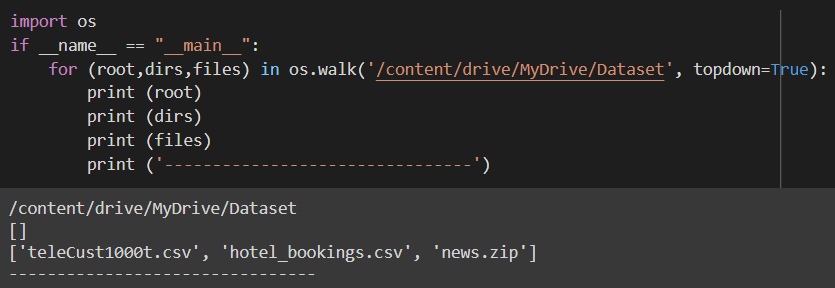##### Math Module in Python

The math module is a built-in Python module that is used for carrying out mathematical operations like – round off, factorial. To utilize the mathematical functions in this module, you must first import them with import math.

###### Example –
```# Factorial calculation
import math
math.factorial(4)
```
###### Output: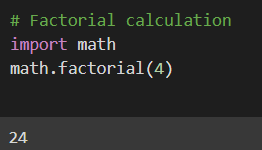Complex data types are not supported by this module. The following is a list of some of the popular functions and characteristics specified in the math module, along with a brief description of what they perform.

 Function Name Use ceil(x) The lowest integer bigger than or equal to x is returned. fabs(x) The absolute value of x is returned. factorial(x) The factorial of x is returned. floor(x) The greatest integer less than or equal to x is returned. fmod(x, y) When x is divided by y, this function returns the residual. isfinite(x) If x is neither infinity nor a NaN, returns True (Not a Number) isinf(x) If x is a positive or negative infinity, returns True. isnan(x) If x is a NaN, this function returns True. Idexp(x, i) x * (2**i) is returned. modf(x) The fractional and integer components of x are returned. trunc(x) The shortened integer value of x is returned. exp(x) e**x is the result. log10(x) The base-10 logarithm of x is returned. pow(x, y) x raised to the power y sqrt(x) square root of x cos(x) The cosine of x is returned. sin(x) The sine of x is returned. tan(x) The tangent of x pi It is a mathematical constant that represents the ratio of a circle’s circumference to its diameter (3.14159…) e Constant with value = 2.71828…
##### Random Numbers in Python

The random module in Python defines a set of functions for generating and manipulating random integers. Random(), a pseudo-random number generator function that generates a random float number between 0.0 and 1.0, is used by functions in the random module. These functions are used in a variety of games, lotteries, and other applications that need the creation of random numbers.

##### Operations on Random Numbers in Python

choice() – The Python programming language has an inbuilt method that returns a random item from a list, tuple, or string. Example –

```import random

# choose a random element from list
lst = [2,4,6,8,10]
print("Random Number :", random.choice(lst))
```
###### Output: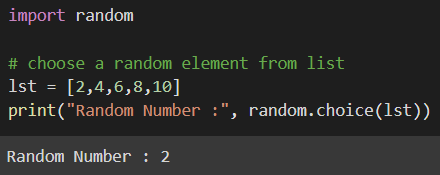random() – This generates a float random integer that is less than 1 and higher than or equal to 0. Example –

```import random

# generate a random number between 0 to 1
print(random.random())
```
###### Output: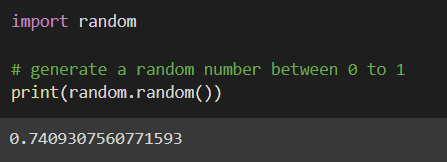randrange(beg, end, step) – The random module has a function called randrange that can produce random numbers from a certain range while also allowing for rooms for steps to be included (). Example –

```import random

# generate random numbers between specified range
print(random.randrange(1, 10, 2))
```
###### Output: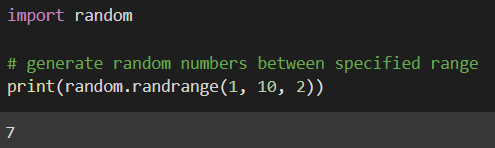##### Dates and Times in Python

Although dates and times aren’t data types in Python, a module named DateTime can be imported to work with both. There is no need to install the Python Datetime module outside because it is included in Python.

The Python Datetime package provides classes for manipulating dates and times. These classes offer a variety of capabilities for working with dates, times, and time intervals. The DateTime module has the following popularly used classes:

date – This is used for getting dates as per the Gregorian calendar. Year, month, and day are its characteristics.

1. You can get the date in year-month-day format by using date()

```# import the date class
from datetime import date

# passing arguments in the
# format year, month, date
my_date = date(2021, 11, 23)

print("Date passed as argument is", my_date)
```
###### Output: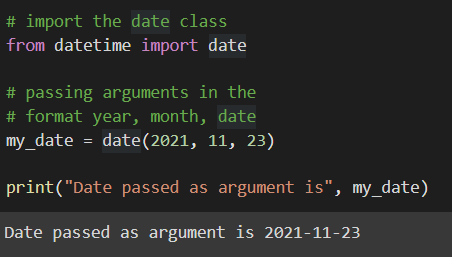2. You can also get the current date by using date.today() function.

```from datetime import date

# calling the today
# function of date class
today = date.today()

print("Today's date is", today)
```
###### Output: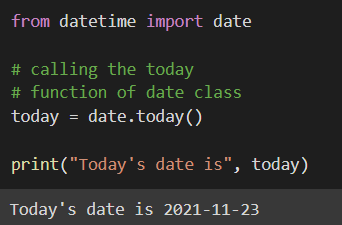time – An idealized time that is independent of any given day and assumes that each day has exactly 24*60*60 seconds. It has the following properties: hour, minute, second, microsecond, and time zone info.

1. You can get the hours, minutes, and seconds of a given time by using Time.hour/ Time.minute/ Time.second

```# extract hours, minutes and seconds
from datetime import time

Time = time(11, 52, 56)

print("hour =", Time.hour)
print("minute =", Time.minute)
print("second =", Time.second)
print("microsecond =", Time.microsecond)
```
###### Output: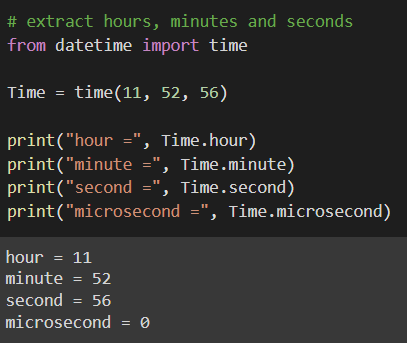2. You can get the time specified in a standard format. First, you need to convert it into a string by using Time.isoformat(). This will convert given hours, minutes, and seconds in the standard format and then into a string.

```# get time in standard format
from datetime import time

Time = time(11, 52, 56)

Str = Time.isoformat()
print("String Representation:", Str)
print(type(Str))
```
###### Output: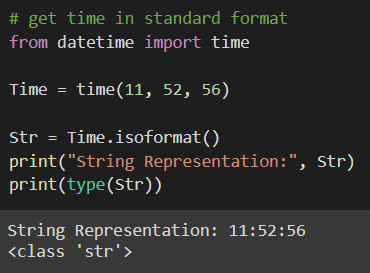datetime – It is a combination of date and time with the attributes year, month, day, hour, minute, second, microsecond, and time zone info.

1. You can get the current date and time by using datetime.now()

```# get current date and time
from datetime import datetime

# Calling now() function
today = datetime.now()

print("Current date and time is", today)
```
###### Output: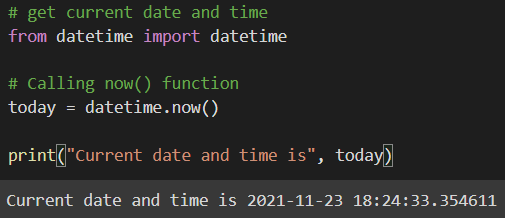In the next article, I am going to discuss Object-Oriented Programming in Python for Data Science with Examples. Here, in this article, I try to explain Regular Expressions and Packages in Python for Data Science with Examples. I hope you enjoy this Regular Expressions and Packages in Python for Data Science article.